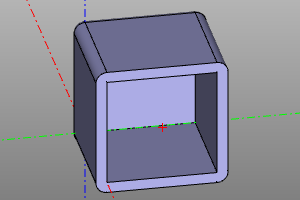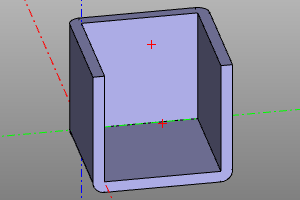# Topologically dependent transformations

There is a class of operations required as part of the model topology. In interactive CAD, we can, using the mouse pointer, point to such an element and point it as a guide. This method is not available in scripted CAD. The general approach of ZenCad is that such an element is specified using the "closest point" method. When specifying an argument, a point is specified instead of a topology element. The selected element is the element, the distance to which from the topology element will be minimal.

## Fillet

Body rounding operation. If the body is solid, the edges are modified. If flat - tops. Fillets are specified by radius `r` and an array of nearest points`refs`. If `refs == None`, all elements of the topology are considered selected.

``````fillet(proto=model, r=radius, refs=referencedPoints)
``````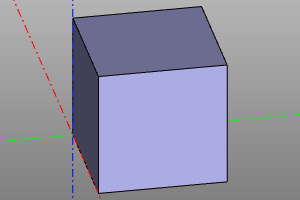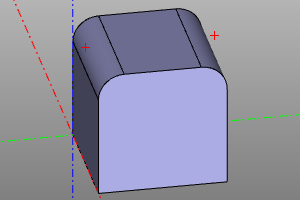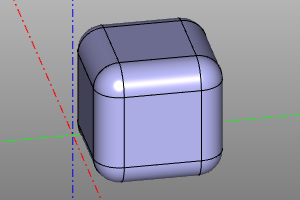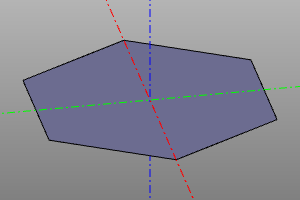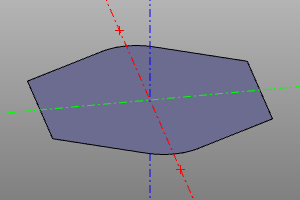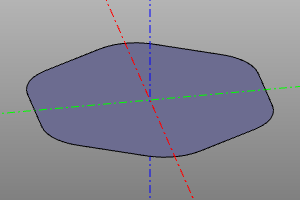## Chamfer

Body chamfering operation. Unlike rounding, it is applied only to solid bodies. The chamfer is specified by the distance `r` taken from the edge to the chamfer line and an array of the nearest points`refs`. If `refs == None`, all elements of the topology are considered selected.

TODO: asymmetrical chamfer.

``````chamfer(proto=model, r=radius, refs=referencedPoints)
``````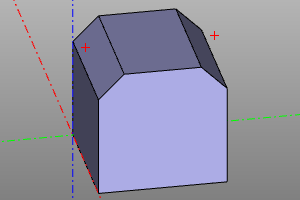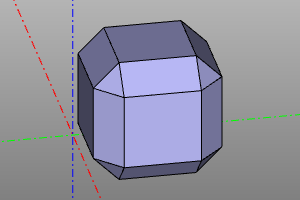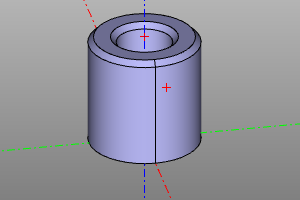## Thicksolid

The operation of creating a thin-walled volumetric body. Defined by the prototype model `shp` and an array of points closest to the removed faces`refs`. The wall thickness `t` is also specified. If the wall thickness is positive, the walls grow outward. If negative - inward.

``````thicksolid(proto=model, t=thickness, refs=referencedPoints)
``````